|

# 地暖专属 大卫23℃纯实木地热地板

随着地暖的快速普及，人们健康家居意识的提高，地热地板尤其是纯实木地热地板，近年来大受市场欢迎，逐渐成为了全球性的趋势。纯实木地热地板是指不改变实木地板自然属性的基础上能直接用于地采暖(地热)环境的实木地板。我们知道，实木地板受潮会膨胀，遇到干燥会收缩，实木地热地板同样会，且当湿度达到一定的极限值时，膨胀系数会大于普通实木。这个不是质量问题，这是木材的天然属性。如何解决“稳定性”这一问题，也成了纯实木地热地板品质的关键。

今天大家不妨随小编一起来看看这款大卫地板23℃纯实木地热：栎木 西贝柳斯吧！

【产品名称】23℃纯实木地热——栎木 西贝柳斯

【产品尺寸】800x85x18mm

【适用场所】适用地热环境

【评测地点】搜狐焦点家居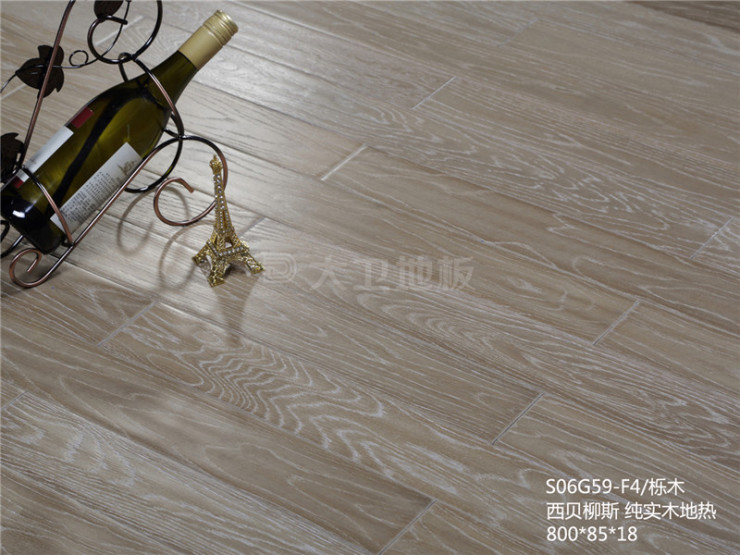稳定性工艺

公司采用“微波热处理”技术，在隔绝氧气的条件下将木材迅速加热温至130-160℃左右、并带压力（0.2MPa）等进行处理。科学除去原木材中的自由水再经高温加压，让木材充分达到其纤维饱点。再对其进行喷湿平衡，让地板的含水量大于其纤维饱和点，表示木材的含水率除吸附水达到饱和外，还有一定数量的自由水。此时，木材如受到干燥或受潮，只是自由水改变，故不会引起湿胀干缩。这样即科学保持实木地板天然木纤维，合理控制原木含水率，与碳化木相比，既保留了木头原有的“活性”，又大大增加了木头的稳定性。不易起翘、不易变形。同样也延长了地板的使用寿命。

漆面材质观察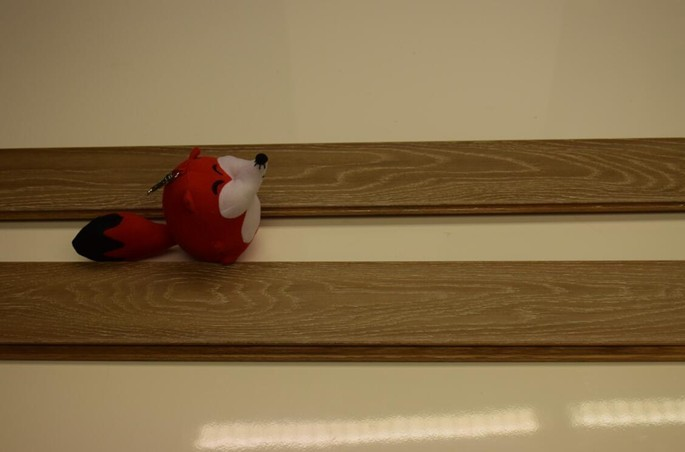这款地板为淡褐色，地板纹路斑驳，如雕刻一般，层次感强。近年来随着简约北欧风格装修的流行，淡色调的地板也更受消费者青睐。而栎木具有明显的构造特征：有光泽、生长年轮明显、环孔材，纹理美丽而独特，弦向纹理庄重大方，径向纹理名贵淡雅，既有其独特的装饰装修效果，又能与多种装修风格匹配。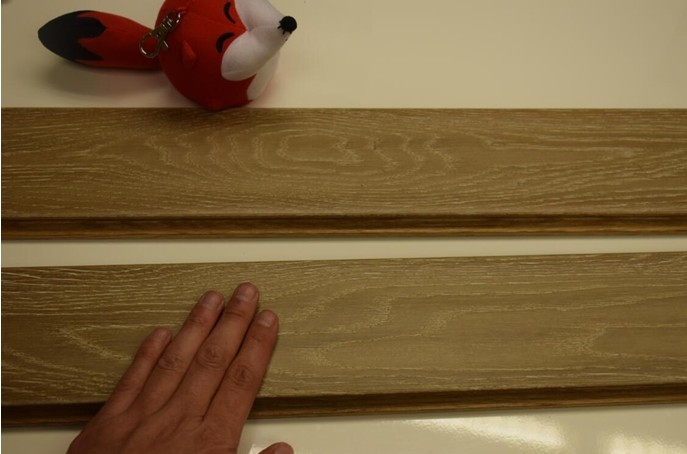地板表面纹理清晰，触摸上去手感温润，隽永斑驳，触感深刻。栎木本身强度大、纹理美观，富有弹性，是上佳的地板用木材树种。仔细观察地板各个边角侧面，各个细节处都非常平滑、整洁、无毛刺，地板做工精良。而地板表面所使用的是高分子UV环保油漆，在纯实木的基础上，保证了后期加工的绿色与环保。大卫油漆工艺及耐磨耗值指标更加严苛，使得地板热传导更快，足下升温，自下而上，调节温度，整体居室温度均匀。

锁扣拼接评测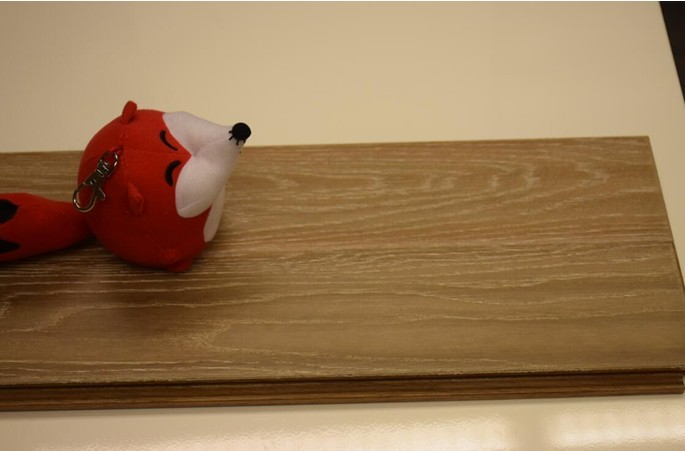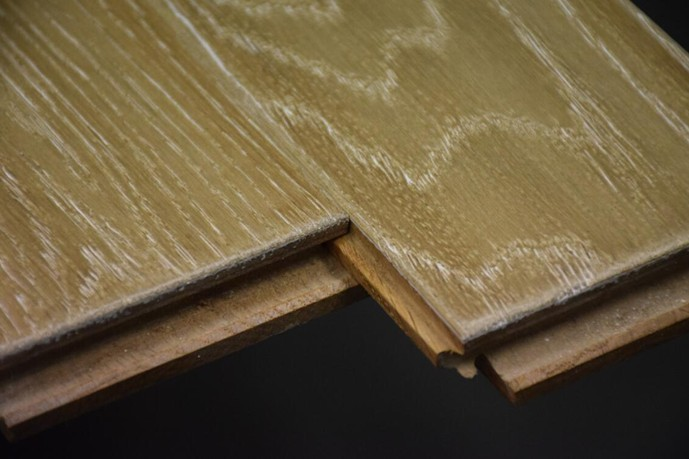地板功能性评测

在地板上挪动家具，小孩乱涂乱画留下污渍，这些在生活中都是不可避免的。一款好的地板，除了外观出众、设计精巧、用材环保以外，更要经得起生活的检验。接下来，搜狐焦点家居将主要从抗污力、防水性、防火阻燃、防滑等几个方面，对这款地板进行评测。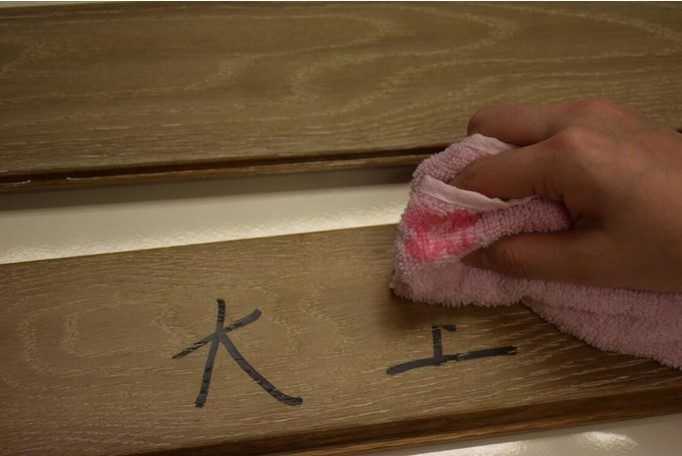使用较难清除的油性笔在地板表面涂写字样，等待数分钟，待字迹略干后，使用湿毛巾擦拭，用一般力道，字迹很快就被擦拭干净。此款地板抗污力非常的棒，平时对生活污渍的打理会比较容易。将地板倾斜着放在台阶上，再让一位成年女性双脚站在地板中间，地板微有弯曲但并未出现断裂现象，可见地板承重力很不错且耐冲击、富于弹性。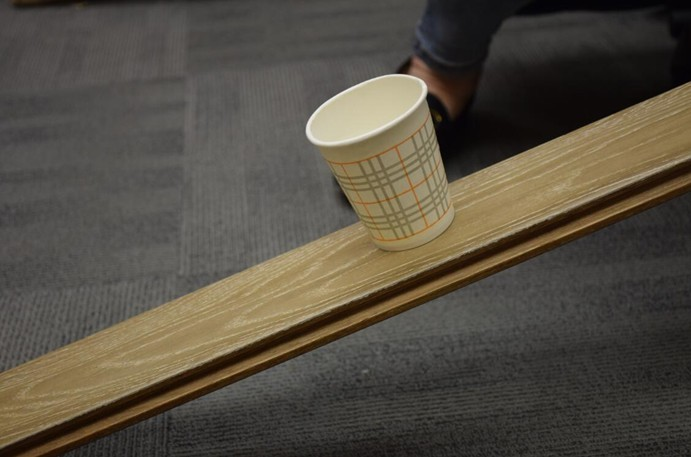将装有装有半杯水的纸杯放在倾斜的地板表面，将地板倾斜度提高至一定倾斜度，以此来测试地板的防滑性能，测试可见纸杯在地板倾斜近30度杯子仍未出现滑落的迹象，地板防滑性不错。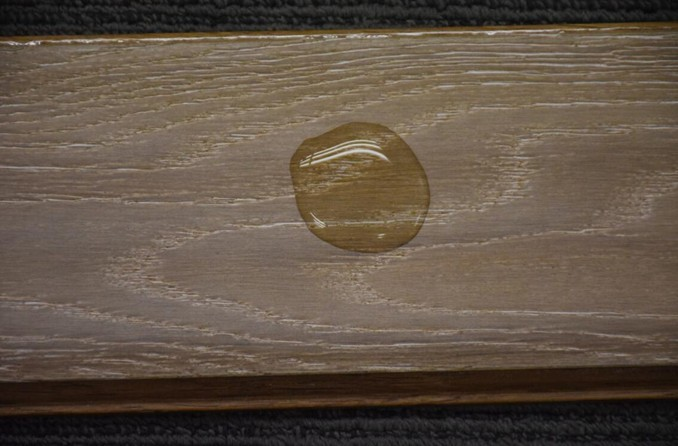小编在地板表面撒上一小摊水，让其静置5分钟左右未见扩散也没有渗透，将地板擦干后，也未见有渗水现象。可见此款地板的表面防水很不错。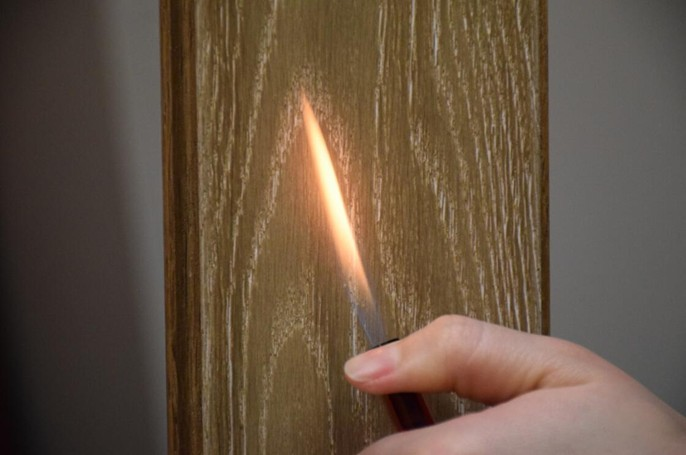打开打火机贴近地板表面灼烧近10秒，地板表面并没有燃烧起来，也未出现起泡现象。用手触摸表面，地板有略有发烫的感觉，这款地板防火阻燃性能很好。

评测总结

栎木素来都是打造宫廷用品的主角，大到帝王宝座、海军舰艇、皇室马车、宫廷家具、宫殿地板，小到店堂雕刻、陈酿葡萄酒的栎木桶等随处可见栎木的踪影。这款大卫地板23℃纯实木地热：S06G59-F4 栎木 西贝柳斯，纹理美观、做工精良，结合微波热处理＆微波烘干技术，具备很强的稳定性，作为地热专属地板热传导更快，足下升温，自下而上，调节温度，使整体居室温度均匀。在耐磨、耐污、承重等测试中，该款产品均表现优异，足以应对日常生活中的考验。而高分子UV环保油漆，宜居自然健康，即使老人、婴儿、孕妇，也可放心使用。

品牌简介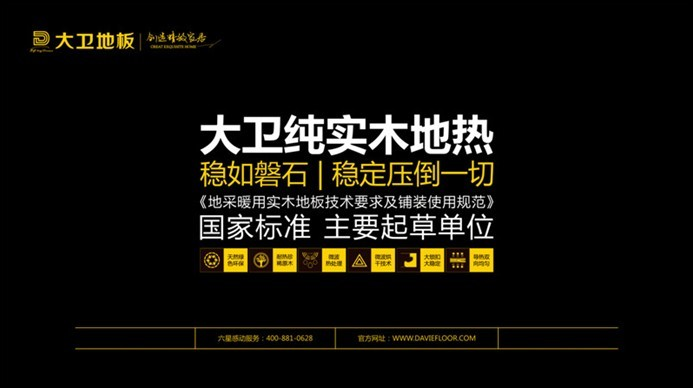大卫，创始于1998年，致力于创造精致家居，是一家专业从事木质家居制造与服务的综合型集团，产品贯穿地板、木门、衣柜、书柜、橱柜、楼梯、酒柜、木饰面、定制家居等九大领域，是国际标准ISO17959:2014《实木地板通用要求》、国家标准《地采暖用实木地板技术要求及铺装使用规范》主要起草单位，荣获中国林业产业突出贡献奖、中国林业产业诚信企业品牌、中国林业产业诚信领军企业、中国林业产业诚信企业-5A等殊荣。

`声明：本文由入驻焦点开放平台的作者撰写，除焦点官方账号外，观点仅代表作者本人，不代表焦点立场错误信息举报电话： 400-099-0099，邮箱：jubao@vip.sohu.com，或点此进行意见反馈，或点此进行举报投诉。`A B C D E F G H J K L M N P Q R S T W X Y Z
A - B - C - D - E
• A
• 鞍山
• 安庆
• 安阳
• 安顺
• 安康
• 澳门
• B
• 北京
• 保定
• 包头
• 巴彦淖尔
• 本溪
• 蚌埠
• 亳州
• 滨州
• 北海
• 百色
• 巴中
• 毕节
• 保山
• 宝鸡
• 白银
• 巴州
• C
• 承德
• 沧州
• 长治
• 赤峰
• 朝阳
• 长春
• 常州
• 滁州
• 池州
• 长沙
• 常德
• 郴州
• 潮州
• 崇左
• 重庆
• 成都
• 楚雄
• 昌都
• 慈溪
• 常熟
• D
• 大同
• 大连
• 丹东
• 大庆
• 东营
• 德州
• 东莞
• 德阳
• 达州
• 大理
• 德宏
• 定西
• 儋州
• 东平
• E
• 鄂尔多斯
• 鄂州
• 恩施
F - G - H - I - J
• F
• 抚顺
• 阜新
• 阜阳
• 福州
• 抚州
• 佛山
• 防城港
• G
• 赣州
• 广州
• 桂林
• 贵港
• 广元
• 广安
• 贵阳
• 固原
• H
• 邯郸
• 衡水
• 呼和浩特
• 呼伦贝尔
• 葫芦岛
• 哈尔滨
• 黑河
• 淮安
• 杭州
• 湖州
• 合肥
• 淮南
• 淮北
• 黄山
• 菏泽
• 鹤壁
• 黄石
• 黄冈
• 衡阳
• 怀化
• 惠州
• 河源
• 贺州
• 河池
• 海口
• 红河
• 汉中
• 海东
• I
• J
• 晋中
• 锦州
• 吉林
• 鸡西
• 佳木斯
• 嘉兴
• 金华
• 景德镇
• 九江
• 吉安
• 济南
• 济宁
• 焦作
• 荆门
• 荆州
• 江门
• 揭阳
• 金昌
• 酒泉
• 嘉峪关
K - L - M - N - P
• K
• 开封
• 昆明
• 昆山
• L
• 廊坊
• 临汾
• 辽阳
• 连云港
• 丽水
• 六安
• 龙岩
• 莱芜
• 临沂
• 聊城
• 洛阳
• 漯河
• 娄底
• 柳州
• 来宾
• 泸州
• 乐山
• 六盘水
• 丽江
• 临沧
• 拉萨
• 林芝
• 兰州
• 陇南
• M
• 牡丹江
• 马鞍山
• 茂名
• 梅州
• 绵阳
• 眉山
• N
• 南京
• 南通
• 宁波
• 南平
• 宁德
• 南昌
• 南阳
• 南宁
• 内江
• 南充
• P
• 盘锦
• 莆田
• 平顶山
• 濮阳
• 攀枝花
• 普洱
• 平凉
Q - R - S - T - W
• Q
• 秦皇岛
• 齐齐哈尔
• 衢州
• 泉州
• 青岛
• 清远
• 钦州
• 黔南
• 曲靖
• 庆阳
• R
• 日照
• 日喀则
• S
• 石家庄
• 沈阳
• 双鸭山
• 绥化
• 上海
• 苏州
• 宿迁
• 绍兴
• 宿州
• 三明
• 上饶
• 三门峡
• 商丘
• 十堰
• 随州
• 邵阳
• 韶关
• 深圳
• 汕头
• 汕尾
• 三亚
• 三沙
• 遂宁
• 山南
• 商洛
• 石嘴山
• T
• 天津
• 唐山
• 太原
• 通辽
• 铁岭
• 泰州
• 台州
• 铜陵
• 泰安
• 铜仁
• 铜川
• 天水
• 天门
• W
• 乌海
• 乌兰察布
• 无锡
• 温州
• 芜湖
• 潍坊
• 威海
• 武汉
• 梧州
• 渭南
• 武威
• 吴忠
• 乌鲁木齐
X - Y - Z
• X
• 邢台
• 徐州
• 宣城
• 厦门
• 新乡
• 许昌
• 信阳
• 襄阳
• 孝感
• 咸宁
• 湘潭
• 湘西
• 西双版纳
• 西安
• 咸阳
• 西宁
• 仙桃
• 西昌
• Y
• 运城
• 营口
• 盐城
• 扬州
• 鹰潭
• 宜春
• 烟台
• 宜昌
• 岳阳
• 益阳
• 永州
• 阳江
• 云浮
• 玉林
• 宜宾
• 雅安
• 玉溪
• 延安
• 榆林
• 银川
• Z
• 张家口
• 镇江
• 舟山
• 漳州
• 淄博
• 枣庄
• 郑州
• 周口
• 驻马店
• 株洲
• 张家界
• 珠海
• 湛江
• 肇庆
• 中山
• 自贡
• 资阳
• 遵义
• 昭通
• 张掖
• 中卫

1室1厅1厨1卫1阳台

1
2
3
4
5

0
1
2

1

1

0
1
2
3报名成功，资料已提交审核A B C D E F G H J K L M N P Q R S T W X Y Z
A - B - C - D - E
• A
• 鞍山
• 安庆
• 安阳
• 安顺
• 安康
• 澳门
• B
• 北京
• 保定
• 包头
• 巴彦淖尔
• 本溪
• 蚌埠
• 亳州
• 滨州
• 北海
• 百色
• 巴中
• 毕节
• 保山
• 宝鸡
• 白银
• 巴州
• C
• 承德
• 沧州
• 长治
• 赤峰
• 朝阳
• 长春
• 常州
• 滁州
• 池州
• 长沙
• 常德
• 郴州
• 潮州
• 崇左
• 重庆
• 成都
• 楚雄
• 昌都
• 慈溪
• 常熟
• D
• 大同
• 大连
• 丹东
• 大庆
• 东营
• 德州
• 东莞
• 德阳
• 达州
• 大理
• 德宏
• 定西
• 儋州
• 东平
• E
• 鄂尔多斯
• 鄂州
• 恩施
F - G - H - I - J
• F
• 抚顺
• 阜新
• 阜阳
• 福州
• 抚州
• 佛山
• 防城港
• G
• 赣州
• 广州
• 桂林
• 贵港
• 广元
• 广安
• 贵阳
• 固原
• H
• 邯郸
• 衡水
• 呼和浩特
• 呼伦贝尔
• 葫芦岛
• 哈尔滨
• 黑河
• 淮安
• 杭州
• 湖州
• 合肥
• 淮南
• 淮北
• 黄山
• 菏泽
• 鹤壁
• 黄石
• 黄冈
• 衡阳
• 怀化
• 惠州
• 河源
• 贺州
• 河池
• 海口
• 红河
• 汉中
• 海东
• I
• J
• 晋中
• 锦州
• 吉林
• 鸡西
• 佳木斯
• 嘉兴
• 金华
• 景德镇
• 九江
• 吉安
• 济南
• 济宁
• 焦作
• 荆门
• 荆州
• 江门
• 揭阳
• 金昌
• 酒泉
• 嘉峪关
K - L - M - N - P
• K
• 开封
• 昆明
• 昆山
• L
• 廊坊
• 临汾
• 辽阳
• 连云港
• 丽水
• 六安
• 龙岩
• 莱芜
• 临沂
• 聊城
• 洛阳
• 漯河
• 娄底
• 柳州
• 来宾
• 泸州
• 乐山
• 六盘水
• 丽江
• 临沧
• 拉萨
• 林芝
• 兰州
• 陇南
• M
• 牡丹江
• 马鞍山
• 茂名
• 梅州
• 绵阳
• 眉山
• N
• 南京
• 南通
• 宁波
• 南平
• 宁德
• 南昌
• 南阳
• 南宁
• 内江
• 南充
• P
• 盘锦
• 莆田
• 平顶山
• 濮阳
• 攀枝花
• 普洱
• 平凉
Q - R - S - T - W
• Q
• 秦皇岛
• 齐齐哈尔
• 衢州
• 泉州
• 青岛
• 清远
• 钦州
• 黔南
• 曲靖
• 庆阳
• R
• 日照
• 日喀则
• S
• 石家庄
• 沈阳
• 双鸭山
• 绥化
• 上海
• 苏州
• 宿迁
• 绍兴
• 宿州
• 三明
• 上饶
• 三门峡
• 商丘
• 十堰
• 随州
• 邵阳
• 韶关
• 深圳
• 汕头
• 汕尾
• 三亚
• 三沙
• 遂宁
• 山南
• 商洛
• 石嘴山
• T
• 天津
• 唐山
• 太原
• 通辽
• 铁岭
• 泰州
• 台州
• 铜陵
• 泰安
• 铜仁
• 铜川
• 天水
• 天门
• W
• 乌海
• 乌兰察布
• 无锡
• 温州
• 芜湖
• 潍坊
• 威海
• 武汉
• 梧州
• 渭南
• 武威
• 吴忠
• 乌鲁木齐
X - Y - Z
• X
• 邢台
• 徐州
• 宣城
• 厦门
• 新乡
• 许昌
• 信阳
• 襄阳
• 孝感
• 咸宁
• 湘潭
• 湘西
• 西双版纳
• 西安
• 咸阳
• 西宁
• 仙桃
• 西昌
• Y
• 运城
• 营口
• 盐城
• 扬州
• 鹰潭
• 宜春
• 烟台
• 宜昌
• 岳阳
• 益阳
• 永州
• 阳江
• 云浮
• 玉林
• 宜宾
• 雅安
• 玉溪
• 延安
• 榆林
• 银川
• Z
• 张家口
• 镇江
• 舟山
• 漳州
• 淄博
• 枣庄
• 郑州
• 周口
• 驻马店
• 株洲
• 张家界
• 珠海
• 湛江
• 肇庆
• 中山
• 自贡
• 资阳
• 遵义
• 昭通
• 张掖
• 中卫• 手机• 分享
• 设计
免费设计
• 计算器
装修计算器
• 入驻
合作入驻
• 联系
联系我们
• 置顶
返回顶部